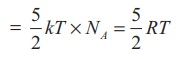Home | | Physics 11th std | Application of law of equipartition energy in specific heat of a gas

# Application of law of equipartition energy in specific heat of a gas

Meyer’s relation Cp − Cv = R connects the two specific heats for one mole of an ideal gas.

Application of law of equipartition energy in specific heat of a gas

Meyer’s relation CP  CV = R connects the two specific heats for one mole of an ideal gas.

Equipartition law of energy is used to calculate the value of CP  CV and the ratio between them γ CP / CV.

Here γ is called adiabatic exponent.

## i)  Monatomic molecule

Average kinetic energy of a moleculeFor one mole, the molar specific heat at constant volume## ii)  Diatomic molecule

Average kinetic energy of a diatomic molecule at low temperature = 5/2kT

Total energy of one mole of gas(Here, the total energy is purely kinetic)

For one mole Specific heat at constant volumeEnergy of a diatomic molecule at high temperature is equal to 7/2RTNote that the CV and CP are higher for diatomic molecules than the mono atomic molecules. It implies that to increase the temperature of diatomic gas molecules by 1°C it require more heat energy than monoatomic molecules.## a)  Linear molecule## b)  Non-linear moleculeNote that according to kinetic theory model of gases the specific heat capacity at constant volume and constant pressure are independent of temperature. But in reality it is not sure. The specific heat capacity varies with the temperature.

### EXAMPLE 9.5

Find the adiabatic exponent γ for mixture of μ 1 moles of monoatomic gas and μ2 moles of a diatomic gas at normal temperature.

### SolutionStudy Material, Lecturing Notes, Assignment, Reference, Wiki description explanation, brief detail
11th Physics : UNIT 9 : Kinetic Theory of Gases : Application of law of equipartition energy in specific heat of a gas |